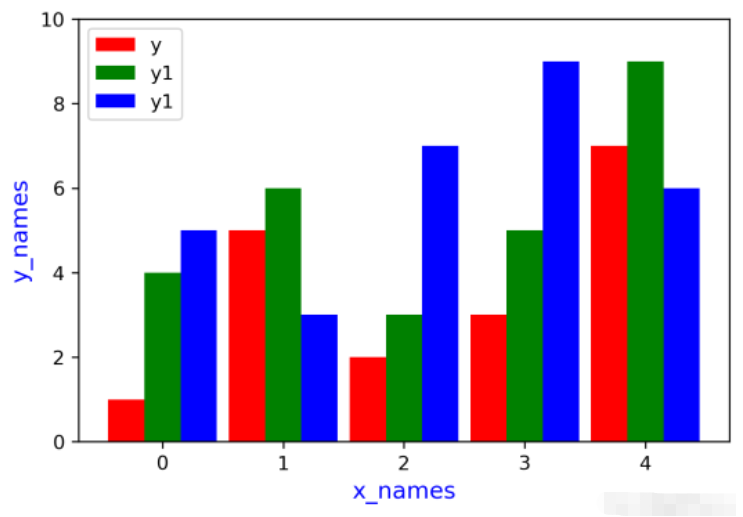# Python怎么绘制多因子柱状图

### 绘图教程

1.打开Spyder软件，我们导入相应的库，以及生成一组数据。（一开始作图的时候，先不要想那么多，跟着敲代码就行）

```#
# 导入相应的库(包)
import numpy as np     # 生成数据的包
import matplotlib.pyplot as plt    #作图的包

# 生成一组数据
x = np.arange(5)
y = [1, 5, 2, 3, 7]
y1 = [4, 6, 3, 5, 9]```

2.查看一下数值

`print(x,y,y1)`3.开始作图，下面是作图代码，照着敲一下，找找感觉，此时图形已经成型

```###
plt.bar(# 设置x和y
x,y,

# 设置柱子宽度
width=0.3,

# 设置柱子颜色
color = "red",

# 设置legend的名称
label = "y")

plt.bar(x+0.3,y1,
width = 0.3,
color = "green",
label = "y1")

# 设置x轴tick的位置
plt.xticks(x+0.3/2,x)

# 显示legend
plt.legend()

# 设置x的标签
plt.xlabel(# x标签的名称
"x_names",

# x标签的字体大小
size = 12,

# x标签的字体颜色
color = "blue")

plt.ylabel("y_names",
size = 12,
color = "blue")

# y轴的范围（x轴的范围只需要将y变为x即可）
plt.ylim(0,10)

# 显示图形
plt.show()```4.目前做的事两列的因子，如果我们有三个，或者多个呢？下面我们假设有三个数据，更改相应的参数后，绘图如下

```# 增加一列数据
y2 = [5,3,7,9,6]

plt.bar(x,y,
width=0.3,
color = "red",
label = "y")
plt.bar(x+0.3,y1,
width = 0.3,
color = "green",
label = "y1")

# 新增绘图部分
plt.bar(x+0.6,y2,
width = 0.3,
color = "blue",
label = "y1")

# 需要更改的部分
plt.xticks(x+0.6/2,x)
plt.legend()

plt.xlabel("x_names",
size = 12,
color = "blue")

plt.ylabel("y_names",
size = 12,
color = "blue")
plt.ylim(0,10)
plt.show()```5.使用hatch参数，可以对柱子进行填充如：hatch = “/”，此外有其他填充形状，如&rsquo;/', &lsquo;&rsquo;, &lsquo;|&rsquo;, &lsquo;-&rsquo;, &lsquo;+&rsquo;, &lsquo;x&rsquo;, &lsquo;o&rsquo;, &lsquo;O&rsquo;, &lsquo;.&rsquo;, &lsquo;*&rsquo;，比如我们使用该参数对柱子进行填充，看看效果

```plt.bar(x,y,
width=0.28,
color = "red",
label = "y",
# 填充形状
hatch = "//")
plt.bar(x+0.3,y1,
width = 0.28,
color = "green",
label = "y1",
# 填充形状
hatch = ".")

plt.bar(x+0.6,y2,
width = 0.28,
color = "blue",
label = "y1",
# 填充形状
hatch = "-")

plt.xticks(x+0.6/2,x)
plt.legend()

plt.xlabel("x_names",
size = 12,
color = "blue")

plt.ylabel("y_names",
size = 12,
color = "blue")
plt.ylim(0,10)
plt.show()```